DraftKings NL Winner Odds
+370
3.7 to 1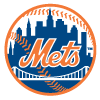Mets
18.3% implied probability

+400
4 to 1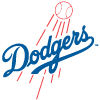Dodgers
17.2% implied probability

+450
4.5 to 1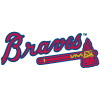Braves
15.7% implied probability

+500
5 to 1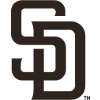Padres
14.4% implied probability

+800
8 to 1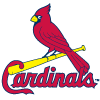Cardinals
9.6% implied probability

+850
8.5 to 1Phillies
9.1% implied probability

+2000
20 to 1Brewers
4.1% implied probability

+2800
28 to 1Giants
3.0% implied probability

+3500
35 to 1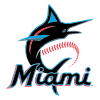Marlins
2.4% implied probability

+4000
40 to 1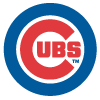Cubs
2.1% implied probability

+6000
60 to 1Diamondbacks
1.4% implied probability

+10000
100 to 1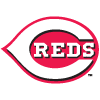Reds
0.9% implied probability

+10000
100 to 1Pirates
0.9% implied probability

+12000
120 to 1Rockies
0.7% implied probability

+20000
200 to 1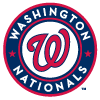Nationals
0.4% implied probability